Command Reference : Matrix Language Reference

 @coldemean
Demean each column of a matrix.
Syntax: @coldemean(m)
m: matrix
Return: matrix
Returns the matrix containing the results from subtracting the column mean from each column of m.
For each element of the output matrix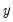: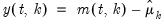for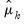the mean of column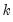where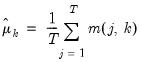(18.1)
where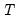is the number of non-missing values in the column. If there are missing values in a column, they are ignored and the number of rows is adjusted.
Examples
matrix m1 = @mnrnd(50, 4)
matrix m1d = @coldemean(m1)
demeans each column of M1 and places the results in M1D.
This operation is equivalent to
vector m1means = @cmeans(m1)
matrix m2d = m1 - @kronecker(@ones(m1.@rows), m1means.@t)
where @cmeans is used to compute the column means of M1.
Cross-references